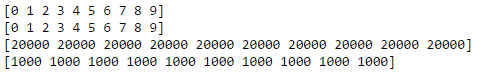Liam

09 Apr, 2016## Summary of 1_notmnist

Basically 1_notmnist is to learn how to display data in Jupyter Notebook. Besides, it also let us know on sklearn - a python machine library - so that we can then compare with TensorFlow. This is the exact ipynb file at Tensorflow Github Repo.

## Notice

This is as a form of sharing and discuss on better way to solve 1_notmnist problem. Do not copy and paste directly as it does not help on improving yourself + the answer is not optimized.

The entire series of TensorFlow Udacity can be found at tensorflow-udacity tag

## Solving Problem 4

``````print(np.unique(train_labels))
print(np.unique(test_labels))
print(np.bincount(train_labels))
print(np.bincount(test_labels))
``````## Comment

Since after shuffling, the image for each count for each train_labels and test_labels is the same, therefore we can say that the data is still good after shuffling!